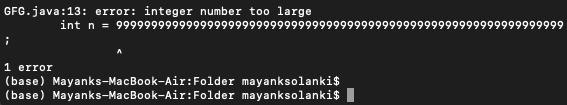GeeksforGeeks App
Open AppBrowser
Continue

# Java Program to Check Whether Number is Divisible by 5

Generic rules in mathematics to test divisibility by 5 in a number system is followed as numbers that end in 5 or 0 are divisible by 5. It doesn’t matter how big the number is.  Modulo operator will be used most frequently in programming when it comes down to divisibility.

Knowledge of the range of numbers that primitive datatypes can store is necessary for the user to get a better understanding while entering custom input as different data types hold a different range of values which is already defined. Here is a quick glimpse of data types that are used more frequently.

Approach:  When it comes down to check divisibility arithmetic operator ‘%’ is used because it returns the remainder of two numbers. Now for a given number be it any random number say ‘n’ be divided by some random number ‘m’ where ‘m’ should be smaller than ‘n’. Let  n=10 and m=3, after using the above approach- n%m that is 10%3 will give remainder equal to unity say it ‘r’ which is obtained when 10 is divided by 3.

Math: 10 % 5 = 0

Example:

Remainder = Dividend % Divisor

1 = 10 % 3

Example:

```Input n    : 464565625
Processing : n % 5 == 0
Output     : Yes

Input n    : 57867456354524524524635602
Processing : n % 5 == 0
Output     : No

Input n    : 346356 56474675364354243220
Processing : n % 5 == 0
Output     : Yes```

Approach 1: Input number is not very large

Let us first assume that the number not very large, we can thus we can take the input as an integer and use the Modulo Arithmetic Operator to check if a number is divisible by 5 or not. Thus, if n % 5 == 0, the number is divisible by 5.

Below is the implementation of the above idea.

## Java

 `// Java program to find if a number``// is divisible by 5 or not` `// importing Classes/Files``import` `java.util.*;` `class` `GFG {` `    ``// Main Driver function``    ``public` `static` `void` `main(String[] args)``    ``{``        ``// Taking any random number to test``        ``int` `n = ``464565625``;` `        ``// Checking if remainder is 0 or not``        ``// when divided by 5``        ``if` `(n % ``5` `== ``0``)` `            ``// Print yes if no is divisible by 5``            ``System.out.println(``"Yes"``);``        ``else` `            ``// Print no if no is not divisible by 5``            ``System.out.println(``"No"``);``    ``}``}`

Output

`Yes`

Time Complexity: O(1)
Auxiliary Space: O(1)

What if the number is very large user hardcoded input. The program will compile but will throw an exception during runtime. There is an issue using the above same approach while dealing with big numbers when it comes downs to the divisibility of big numbers as the existing data types discussed above has a specific size over which the range is fixed for what they can store. If the above approach is used where data types are forced to store big integers in primitive data types error is thrown at the compile time itself: ‘Integer number too large.’Approach 2: Input number is not very large

• Use Java Big Integers and check divisibility by using modulo operator, similar to the above mentioned.
• Use the fact that a number is divisible by 5 if its last digit is either 0 or 5.

Using the above-mentioned fact we just have to check if the last digit is 0 or 5. Below is the implementation of the above idea.

## Java

 `// Java program to find if a number``// is divisible by 5 or not` `// Importing Classes/Files``import` `java.util.*;` `public` `class` `GFG {` `    ``// Function for divisibility by 5``    ``static` `boolean` `isDivisibleBy5(String num)``    ``{``        ``// Number of Digits``        ``int` `sz = num.length();` `        ``// Checking if last digit is 5``        ``// Checking if last digit is 0``        ``return` `(``            ``(Integer.parseInt(num.substring(sz - ``1``)) == ``5``)``            ``|| (Integer.parseInt(num.substring(sz - ``1``))``                ``== ``0``));``    ``}` `    ``// Main Driver function``    ``public` `static` `void` `main(String[] args)``    ``{``        ``// Considering any big random number``        ``String num = ``"464565625"``;` `        ``// Condition check for divisibility``        ``if` `(isDivisibleBy5(num))` `            ``// Print yes if no is divisible``            ``System.out.println(``"Yes"``);``        ``else` `            ``// Print no if no is not divisible``            ``System.out.println(``"No"``);``    ``}``}`

Output

`Yes`

Time Complexity: O(1)
Auxiliary Space: O(1)

My Personal Notes arrow_drop_up
Related Tutorials